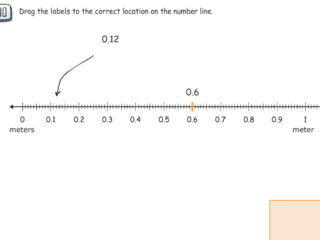# Interactive Problem Bank

•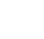Fraction Circles
•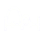Number Lines
•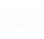Fraction Bars
•Sets
•Area Models
•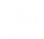Base Ten Blocks

## Decimals on the Number Line

Students locate and name tenths and hundredths on the number line using visual models, place value, and reasoning about relative size.

### Decomposing Decimals into Fractions on the Number Line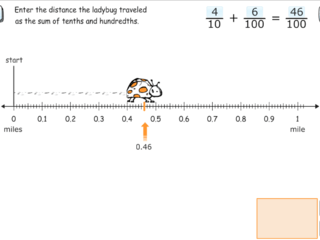### Estimating Decimals on the Number Line### Comparing Decimals on the Number Line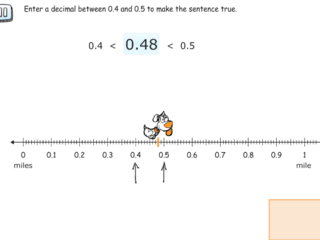### Ordering Decimals on the Number Line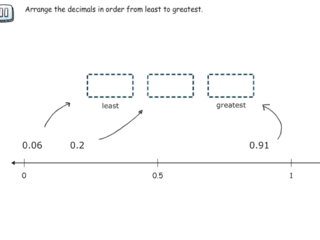### Locating Tenths on the Number Line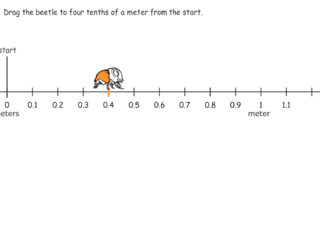### Measuring Tenths on the Number Line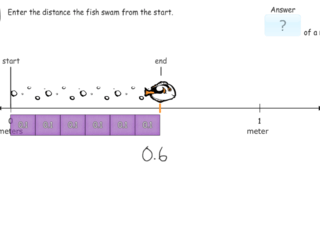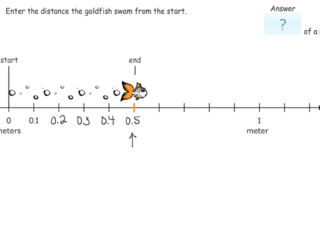### Labeling Tenths on the Number Line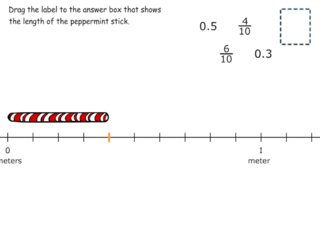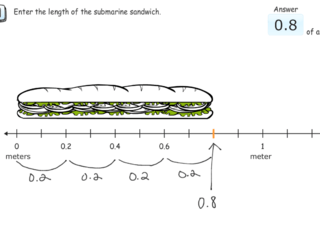### Composing Decimals on the Number Line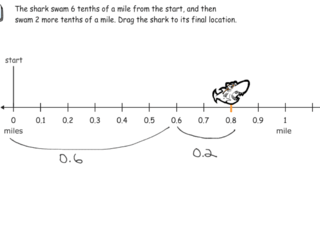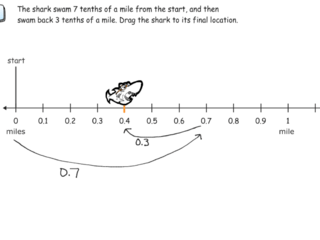### Locating Hundredths on the Number Line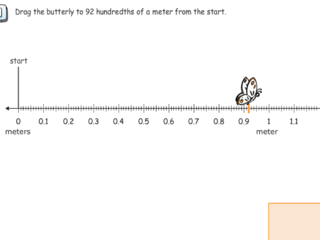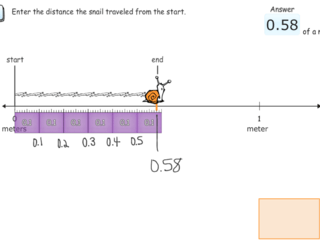### Labeling Hundredths on the Number Line